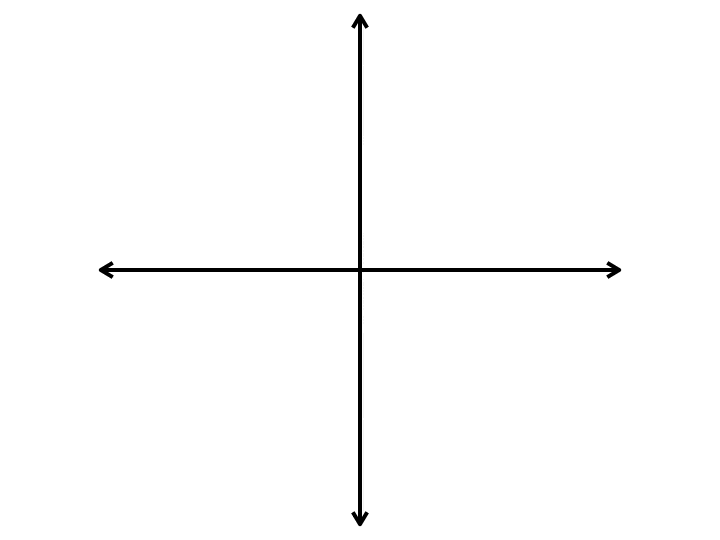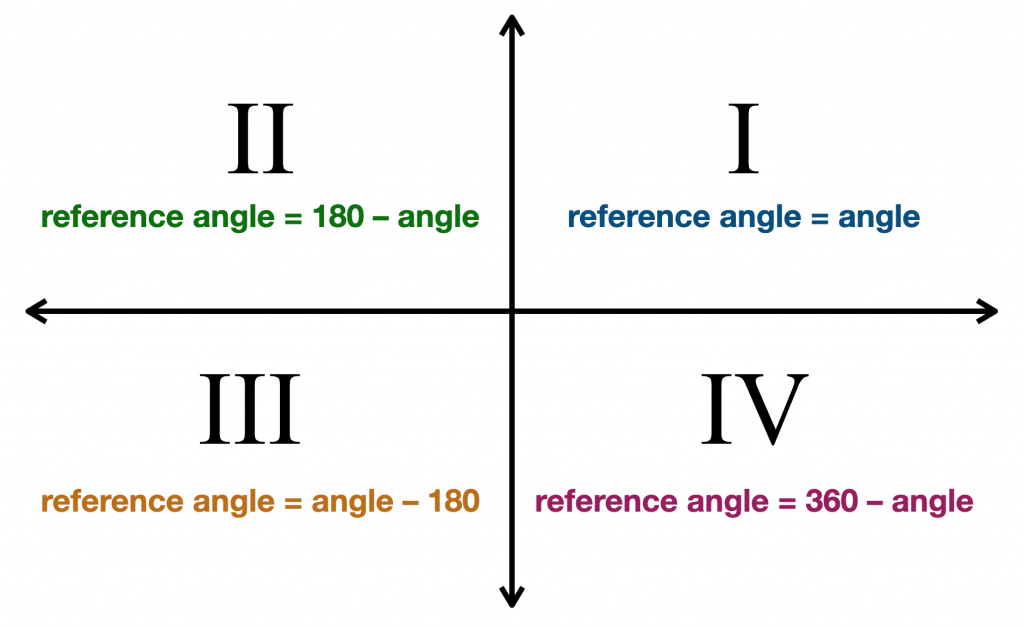# Reference Angle Calculator

To use the reference angle calculator, simply enter any angle into the angle box to find its reference angle, which is the acute angle that corresponds to the angle entered. The calculator automatically applies the rules we’ll review below.

Go back to Calculators page

## What is a Reference Angle, anyway?

Imagine a coordinate plane. Let’s say we want to draw an angle that’s 144° on our plane. We start on the right side of the x-axis, where three o’clock is on a clock. We rotate counterclockwise, which starts by moving up. We keep going past the 90° point (the top part of the y-axis) until we get to 144°. We draw a ray from the origin, which is the center of the plane, to that point. Now we have a ray that we call the terminal side. But we need to draw one more ray to make an angle. We have a choice at this point. Our second ray needs to be on the x-axis. If we draw it from the origin to the right side, we’ll have drawn an angle that measures 144°. If we draw it to the left, we’ll have drawn an angle that measures 36°. This second angle is the reference angle. It’s always the smaller of the two angles, will always be less than or equal to 90°, and it will always be positive. Here’s an animation that shows a reference angle for four different angles, each of which is in a different quadrant. Notice how the second ray is always on the x-axis.## How is the Reference Angle Useful?

The reference angle always has the same trig function values as the original angle. Notice the word values there. The sign may not be the same, but the value always will be. This is useful for common angles like 45° and 60° that we will encounter over and over again. Once we know their sine, cosine, and tangent values, we also know the values for any angle whose reference angle is also 45° or 60°. As for the sign, remember that Sine is positive in the 1st and 2nd quadrant and Cosine is positive in the 1st and 4th quadrant.## How do We Find the Reference Angle without a Calculator?

How we find the reference angle depends on the quadrant of the terminal side.

When the terminal side is in the first quadrant (angles from 0° to 90°), our reference angle is the same as our given angle. This makes sense, since all the angles in the first quadrant are less than 90°. So, if our given angle is 33°, then its reference angle is also 33°.

When the terminal side is in the second quadrant (angles from 90° to 180°), our reference angle is 180° minus our given angle. So, if our given angle is 110°, then its reference angle is 180° – 110° = 70°.

When the terminal side is in the third quadrant (angles from 180° to 270°), our reference angle is our given angle minus 180°. So, if our given angle is 214°, then its reference angle is 214° – 180° = 34°.

When the terminal side is in the fourth quadrant (angles from 270° to 360°), our reference angle is 360° minus our given angle. So, if our given angle is 332°, then its reference angle is 360° – 332° = 28°.## What if Our Angle is Greater than 360°?

When an angle is greater than 360°, that means it has rotated all the way around the coordinate plane and kept on going. In order to find its reference angle, we first need to find its corresponding angle between 0° and 360°. This is easy to do. We just keep subtracting 360 from it until it’s below 360. For instance, if our angle is 544°, we would subtract 360° from it to get 184° (544° – 360° = 184°). Now we would notice that it’s in the third quadrant, so we’d subtract 180° from it to find that our reference angle is 4°.

## What if Our Angle is Negative?

When an angle is negative, we move the other direction to find our terminal side. This means we move clockwise instead of counterclockwise when drawing it. Or we can calculate it by simply adding it to 360°. For instance, if our given angle is –110°, then we would add it to 360° to find our positive angle of 250° (–110° + 360° = 250°). Now we would have to see that we’re in the third quadrant and apply that rule to find our reference angle (250° – 180° = 70°).

This calculator can quickly find the reference angle, but in a pinch, remember that a quick sketch can help you remember the rules for calculating the reference angle in each quadrant.